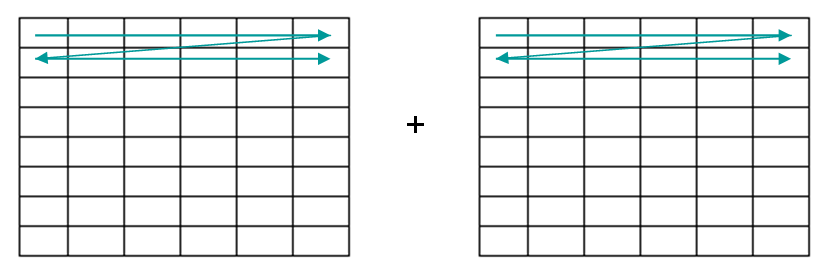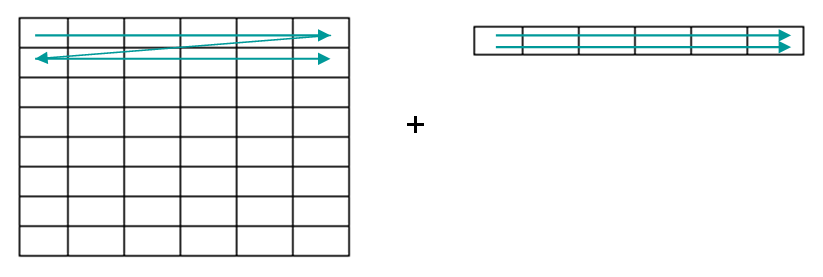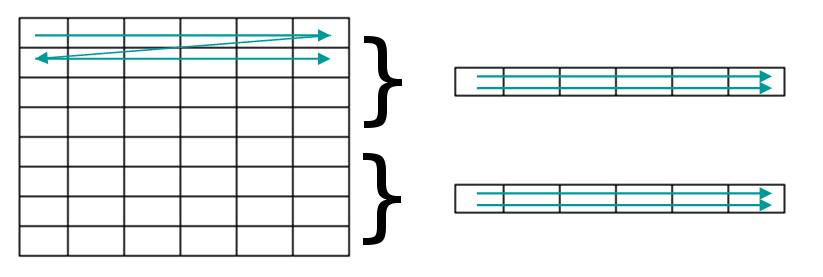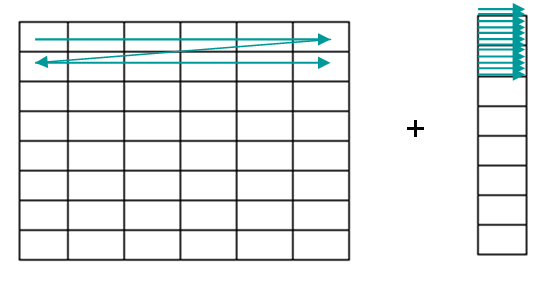$$\renewcommand\AA{\unicode{x212B}}$$

# Multiply v1¶

## Summary¶

The Multiply algorithm will multiply the data values and calculate the corresponding error values of two compatible workspaces.

## Properties¶

Name

Direction

Type

Default

Description

LHSWorkspace

Input

MatrixWorkspace

Mandatory

The name of the input workspace on the left hand side of the operation

RHSWorkspace

Input

MatrixWorkspace

Mandatory

The name of the input workspace on the right hand side of the operation

OutputWorkspace

Output

MatrixWorkspace

Mandatory

The name to call the output workspace

AllowDifferentNumberSpectra

Input

boolean

False

Are workspaces with different number of spectra allowed? For example, the LHSWorkspace might have one spectrum per detector, but the RHSWorkspace could have its spectra averaged per bank. If true, then matching between the LHS and RHS spectra is performed (all detectors in a LHS spectrum have to be in the corresponding RHS) in order to apply the RHS spectrum to the LHS.

ClearRHSWorkspace

Input

boolean

False

For EventWorkspaces only. This will clear out event lists from the RHS workspace as the binary operation is applied. This can prevent excessive memory use, e.g. when subtracting an EventWorkspace from another: memory use will be approximately constant instead of increasing by 50%. At completion, the RHS workspace will be empty.

## Description¶

OutputWorkspace = LHSWorkspace * RHSWorkspace

The algorithm will perform the * operation on the data and associated errors from any two compatible workspaces. Workspaces are compatible if:

• The sizes of the two workspaces are compatible (see below)

• If the two workspaces contain Y bins then the values in these must be identical

• the units of the axes match

• the distribution status/counts units match

For information about how errors are handled and propagated see Error Propagation.

### Compatible Sizes¶

 Workspaces are compatible if they are identically sized in which case the values of each cell within each histogram are multiplied individually. The green arrows shows the order in which the data is multiplied.They are also compatible if they match the size dimension horizontally, in which case the same values are multiplied by each histogram in the workspace.If AllowDifferentNumberSpectra is True, then the LHS and RHS spectra are matched using detector IDs. The corresponding spectra are multiplied by the matching counterpart.If they match in size vertically then the data values will be multiplied by each bin of the histogram.Finally a workspace containing a single value is compatible to any workspace, in which case the same value will be multiplied uniformly by every bin in each histogram.

### Operations on EventWorkspaces vs Workspace2Ds¶

LHS

*

RHS

=

Output

Notes

Workspace2D

*

Workspace2D

=

Workspace2D

The number of bins DO need to match for both operands.

Workspace2D

*

EventWorkspace

=

Workspace2D

The histogram representation of the RHS EventWorkspace is used as the right-hand operand. The number of bins DO need to match for both operands.

EventWorkspace

*

EventWorkspace

=

EventWorkspace

The histogram representation of the RHS EventWorkspace is used as the right-hand operand. The number of bins DO NOT need to match for both operands. The output EventWorkspace will use WeightedEvents, where each event’s weight will have been multiplied by the Y value at the corresponding X bin on the RHS.

EventWorkspace

*

Workspace2D

=

EventWorkspace

The histogram representation of the RHS EventWorkspace is used as the right-hand operand. The number of bins DO NOT need to match for both operands.

EventWorkspace

*

WorkspaceSingleValue

=

EventWorkspace

The value (and error) is applied to the weight of every event in the workspace. The output EventWorkspace will use WeightedEvents. If Output–LHS, then the operation is performed in-place and no copying of workspaces is done.

## Usage¶

Example - Multiply as an algorithm

# create histogram workspaces
dataX1 = [0,1,2,3,4,5,6,7,8,9] # or use dataX1=range(0,10)
dataY1 = [0,1,2,3,4,5,6,7,8] # or use dataY1=range(0,9)
dataE1 = [0,1,2,3,4,5,6,7,8] # or use dataE1=range(0,9)
dataX2 = [0,1,2,3,4,5,6,7,8,9] #X-values must be identical
dataY2 = [2,2,2,2,2,2,2,2,2]
dataE2 = [3,3,3,3,3,3,3,3,3]
ws1 = CreateWorkspace(dataX1, dataY1, dataE1)
ws2 = CreateWorkspace(dataX2, dataY2, dataE2)

# perform the algorithm
ws = Multiply(ws1, ws2)

print("The updated Error values are: {}".format(ws.readE(0)))


Output:

The X values are: [ 0.  1.  2.  3.  4.  5.  6.  7.  8.  9.]
The Y values are: [  0.   2.   4.   6.   8.  10.  12.  14.  16.]
The updated Error values are: [  0.           3.60555128   7.21110255  10.81665383  14.4222051
18.02775638  21.63330765  25.23885893  28.8444102 ]


Example - Multiply as an operator

# create histogram workspaces
dataX1 = [0,1,2,3,4,5,6,7,8,9] # or use dataX1=range(0,10)
dataY1 = [0,1,2,3,4,5,6,7,8] # or use dataY1=range(0,9)
dataE1 = [0,1,2,3,4,5,6,7,8] # or use dataE1=range(0,9)
dataX2 = [0,1,2,3,4,5,6,7,8,9] #X-values must be identical
dataY2 = [2,2,2,2,2,2,2,2,2]
dataE2 = [3,3,3,3,3,3,3,3,3]
ws1 = CreateWorkspace(dataX1, dataY1, dataE1)
ws2 = CreateWorkspace(dataX2, dataY2, dataE2)

# perform the algorithm
ws = ws1 * ws2

print("The updated Error values are: {}".format(ws.readE(0)))


Output:

The X values are: [ 0.  1.  2.  3.  4.  5.  6.  7.  8.  9.]
The Y values are: [  0.   2.   4.   6.   8.  10.  12.  14.  16.]
The updated Error values are: [  0.           3.60555128   7.21110255  10.81665383  14.4222051
18.02775638  21.63330765  25.23885893  28.8444102 ]


Example - Multiply using in-place operator

# create histogram workspaces
dataX1 = [0,1,2,3,4,5,6,7,8,9] # or use dataX1=range(0,10)
dataY1 = [0,1,2,3,4,5,6,7,8] # or use dataY1=range(0,9)
dataE1 = [0,1,2,3,4,5,6,7,8] # or use dataE1=range(0,9)
dataX2 = [0,1,2,3,4,5,6,7,8,9] #X-values must be identical
dataY2 = [2,2,2,2,2,2,2,2,2]
dataE2 = [3,3,3,3,3,3,3,3,3]
ws = CreateWorkspace(dataX1, dataY1, dataE1)
ws1 = CreateWorkspace(dataX2, dataY2, dataE2)

# perform the algorithm
ws *= ws1

print("The updated Error values are: {}".format(ws.readE(0)))


Output:

The X values are: [ 0.  1.  2.  3.  4.  5.  6.  7.  8.  9.]
The Y values are: [  0.   2.   4.   6.   8.  10.  12.  14.  16.]
The updated Error values are: [  0.           3.60555128   7.21110255  10.81665383  14.4222051
18.02775638  21.63330765  25.23885893  28.8444102 ]


Example - Multiply with a Scalar

# create histogram workspaces
dataX1 = [0,1,2,3,4,5,6,7,8,9] # or use dataX1=range(0,10)
dataY1 = [0,1,2,3,4,5,6,7,8] # or use dataY1=range(0,9)
dataE1 = [0,1,2,3,4,5,6,7,8] # or use dataE1=range(0,9)
ws1 = CreateWorkspace(dataX1, dataY1, dataE1)

# perform the algorithm
ws = ws1 * 2.5

print("The updated Error values are: {}".format(ws.readE(0)))


Output:

The X values are: [ 0.  1.  2.  3.  4.  5.  6.  7.  8.  9.]
The Y values are: [  0.    2.5   5.    7.5  10.   12.5  15.   17.5  20. ]
The updated Error values are: [  0.    2.5   5.    7.5  10.   12.5  15.   17.5  20. ]


Categories: AlgorithmIndex | Arithmetic